# The sinc-Galerkin method and its applications on singular Dirichlet-type boundary value problems

## Abstract

The application of the sinc-Galerkin method to an approximate solution of second-order singular Dirichlet-type boundary value problems were discussed in this study. The method is based on approximating functions and their derivatives by using the Whittaker cardinal function. The differential equation is reduced to a system of algebraic equations via new accurate explicit approximations of the inner products without any numerical integration which is needed to solve matrix system. This study shows that the sinc-Galerkin method is a very effective and powerful tool in solving such problems numerically. At the end of the paper, the method was tested on several examples with second-order Dirichlet-type boundary value problems.

## 1 Introduction

Sinc methods were introduced by Frank Stenger in  and expanded upon by him in . Sinc functions were first analyzed in  and . An extensive research of sinc methods for two-point boundary value problems can be found in [5, 6]. In [7, 8], parabolic and hyperbolic problems were discussed in detail. Some kind of singular elliptic problems were solved in , and the symmetric sinc-Galerkin method was introduced in . Sinc domain decomposition was presented in  and . Iterative methods for symmetric sinc-Galerkin systems were discussed in [15, 16] and . Sinc methods were discussed thoroughly in . Applications of sinc methods can also be found in [19, 20] and . The article  summarizes the results obtained to date on sinc numerical methods of computation. In , a numerical solution of a Volterra integro-differential equation by means of the sinc collocation method was considered. The paper  illustrates the application of a sinc-Galerkin method to an approximate solution of linear and nonlinear second-order ordinary differential equations, and to an approximate solution of some linear elliptic and parabolic partial differential equations in the plane. The fully sinc-Galerkin method was developed for a family of complex-valued partial differential equations with time-dependent boundary conditions . Some novel procedures of using sinc methods to compute solutions to three types of medical problems were illustrated in , and sinc-based algorithm was used to solve a nonlinear set of partial differential equations in . A new sinc-Galerkin method was developed for approximating the solution of convection diffusion equations with mixed boundary conditions on half-infinite intervals in . The work which was presented in  deals with the sinc-Galerkin method for solving nonlinear fourth-order differential equations with homogeneous and nonhomogeneous boundary conditions. In , sinc methods were used to solve second-order ordinary differential equations with homogeneous Dirichlet-type boundary conditions.

## 2 Sinc functions preliminaries

Let C denote the set of all complex numbers, and for all $z\in C$, define the sine cardinal or sinc function by

$sinc\left(z\right)=\left\{\begin{array}{cc}\frac{sin\left(\pi z\right)}{\pi z},\hfill & y\ne 0,\hfill \\ 1,\hfill & y=0.\hfill \end{array}$
(2.1)

For $h>0$, the translated sinc function with evenly spaced nodes is given by

$sinc\left(k,h\right)\left(z\right)=\left\{\begin{array}{cc}\frac{sin\left(\pi \frac{z-kh}{h}\right)}{\pi \frac{z-kh}{h}},\hfill & z\ne kh,\hfill \\ 1,\hfill & z=kh.\hfill \end{array}$
(2.2)

For various values of k, the sinc basis function $S\left(k,\pi /4\right)\left(x\right)$ on the whole real line $-\mathrm{\infty } is illustrated in Figure 1. For various values of h, the central function $S\left(0,h\right)\left(x\right)$ is illustrated in Figure 2.

If a function $f\left(x\right)$ is defined over the real line, then for $h>0$, the series

$C\left(f,h\right)\left(x\right)=\sum _{k=-\mathrm{\infty }}^{\mathrm{\infty }}f\left(kh\right)sinc\left(\frac{x-kh}{h}\right)$
(2.3)

is called the Whittaker cardinal expansion of f whenever this series converges. The infinite strip ${D}_{s}$ of the complex w plane, where $d>0$, is given by

${D}_{s}\equiv \left\{w=u+iv:|v|
(2.4)

In general, approximations can be constructed for infinite, semi-infinite and finite intervals. Define the function

$w=\varphi \left(z\right)=ln\left(\frac{z}{1-z}\right)$
(2.5)

which is a conformal mapping from ${D}_{E}$, the eye-shaped domain in the z-plane, onto the infinite strip ${D}_{S}$, where

${D}_{E}=z=\left\{x+iy:|arg\left(\frac{z}{1-z}\right)|
(2.6)

This is shown in Figure 3.

For the sinc-Galerkin method, the basis functions are derived from the composite translated sinc functions

${S}_{h}\left(z\right)=S\left(k,h\right)\left(z\right)=sinc\left(\frac{\varphi \left(z\right)-kh}{h}\right)$
(2.7)

for $z\in {D}_{E}$. These are shown in Figure 4 for real values x. The function $z={\varphi }^{-1}\left(w\right)=\frac{{e}^{w}}{1+{e}^{w}}$ is an inverse mapping of $w=\varphi \left(z\right)$. We may define the range of ${\varphi }^{-1}$ on the real line as

$\mathrm{\Gamma }=\left\{{\varphi }^{-1}\left(u\right)\in {D}_{E}:-\mathrm{\infty }
(2.8)

the evenly spaced nodes ${\left\{kh\right\}}_{k=-\mathrm{\infty }}^{\mathrm{\infty }}$ on the real line. The image which corresponds to these nodes is denoted by

${x}_{k}={\varphi }^{-1}\left(kh\right)=\frac{{e}^{kh}}{1+{e}^{kh}}.$
(2.9)

A list of conformal mappings may be found in Table 1 .

Definition 2.1 Let ${D}_{E}$ be a simply connected domain in the complex plane C, and let $\partial {D}_{E}$ denote the boundary of ${D}_{E}$. Let a, b be points on $\partial {D}_{E}$ and ϕ be a conformal map ${D}_{E}$ onto ${D}_{S}$ such that $\varphi \left(a\right)=-\mathrm{\infty }$ and $\varphi \left(b\right)=-\mathrm{\infty }$. If the inverse map of ϕ is denoted by φ, define

$\mathrm{\Gamma }=\left\{{\varphi }^{-1}\left(u\right)\in {D}_{E}:-\mathrm{\infty }
(2.10)

and ${z}_{k}=\phi \left(kh\right)$, $k=\mp 1,\mp 2,\dots$ .

Definition 2.2 Let $B\left({D}_{E}\right)$ be the class of functions F that are analytic in ${D}_{E}$ and satisfy

(2.11)

where

$L=\left\{iy:|y|
(2.12)

and those on the boundary of ${D}_{E}$ satisfy

$T\left(F\right)={\int }_{\partial {D}_{E}}|F\left(z\right)\phantom{\rule{0.2em}{0ex}}dz|<\mathrm{\infty }.$
(2.13)

The proof of following theorems can be found in .

Theorem 2.1 Let Γ be $\left(0,1\right)$, $F\in B\left({D}_{E}\right)$, then for $h>0$ sufficiently small,

${\int }_{\mathrm{\Gamma }}F\left(z\right)\phantom{\rule{0.2em}{0ex}}dz-h\sum _{j=-\mathrm{\infty }}^{\mathrm{\infty }}\frac{F\left({z}_{j}\right)}{{\varphi }^{\prime }\left({z}_{j}\right)}=\frac{i}{2}{\int }_{\partial D}\frac{F\left(z\right)k\left(\varphi ,h\right)\left(z\right)}{sin\left(\pi \varphi \left(z\right)/h\right)}\phantom{\rule{0.2em}{0ex}}dz\equiv {I}_{F},$
(2.14)

where

$|k\left(\varphi ,h\right){|}_{z\in \partial D}=|{e}^{\left[\frac{i\pi \varphi \left(z\right)}{h}sgn\left(Im\varphi \left(z\right)\right)\right]}{|}_{z\in \partial D}={e}^{\frac{-\pi d}{h}}.$
(2.15)

For the sinc-Galerkin method, the infinite quadrature rule must be truncated to a finite sum. The following theorem indicates the conditions under which an exponential convergence results.

Theorem 2.2 If there exist positive constants α, β and C such that

$|\frac{F\left(x\right)}{{\varphi }^{\prime }\left(x\right)}|\le C\left\{\begin{array}{cc}{e}^{-\alpha |\varphi \left(x\right)|},\hfill & x\in \psi \left(\left(-\mathrm{\infty },\mathrm{\infty }\right)\right),\hfill \\ {e}^{-\beta |\varphi \left(x\right)|},\hfill & x\in \psi \left(\left(0,\mathrm{\infty }\right)\right),\hfill \end{array}$
(2.16)

then the error bound for the quadrature rule (2.14) is

$|{\int }_{\mathrm{\Gamma }}F\left(x\right)\phantom{\rule{0.2em}{0ex}}dx-h\sum _{j=-N}^{N}\frac{F\left({x}_{j}\right)}{{\varphi }^{\prime }\left({x}_{j}\right)}|\le C\left(\frac{{e}^{-\alpha Nh}}{\alpha }+\frac{{e}^{-\beta Nh}}{\beta }\right)+|{I}_{F}|.$
(2.17)

The infinite sum in (2.14) is truncated with the use of (2.16) to arrive at the inequality (2.17). Making the selections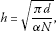(2.18)(2.19)

where $〚\cdot 〛$ is an integer part of the statement and N is the integer value which specifies the grid size, then

${\int }_{\mathrm{\Gamma }}F\left(x\right)\phantom{\rule{0.2em}{0ex}}dx=h\sum _{j=-N}^{N}\frac{F\left({x}_{j}\right)}{{\varphi }^{\prime }\left({x}_{j}\right)}+O\left({e}^{-{\left(\pi \alpha \phantom{\rule{0.2em}{0ex}}dN\right)}^{1/2}}\right).$
(2.20)

We used Theorems 2.1 and 2.2 to approximate the integrals that arise in the formulation of the discrete systems corresponding to a second-order boundary value problem.

Theorem 2.3 Let ϕ be a conformal one-to-one map of the simply connected domain ${D}_{E}$ onto ${D}_{S}$. Then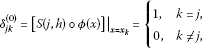(2.21)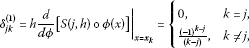(2.22)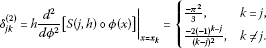(2.23)

## 3 The sinc-Galerkin method for singular Dirichlet-type boundary value problems

Consider the following problem:

${y}^{″}+P\left(x\right){y}^{\prime }+Q\left(x\right)y=F\left(x\right)$
(3.1)

with Dirichlet-type boundary condition

$y\left(a\right)=0,\phantom{\rule{2em}{0ex}}y\left(b\right)=0,$
(3.2)

where P, Q and F are analytic on D. We consider sinc approximation by the formula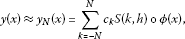(3.3)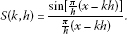(3.4)

The unknown coefficients ${c}_{k}$ in Eq. (3.3) are determined by orthogonalizing the residual with respect to the sinc basis functions. The Galerkin method enables us to determine the ${c}_{k}$ coefficients by solving the linear system of equations

$〈L{y}_{N}-F,S\left(k,h\right)\circ \varphi \left(x\right)〉=0,\phantom{\rule{1em}{0ex}}k=-N,-N+1,\dots ,N-1,N.$
(3.5)

Let ${f}_{1}$ and ${f}_{2}$ be analytic functions on D and the inner product in (3.5) be defined as follows:

$〈{f}_{1},{f}_{2}〉={\int }_{\mathrm{\Gamma }}w\left(x\right){f}_{1}\left(x\right){f}_{2}\left(x\right)\phantom{\rule{0.2em}{0ex}}dx,$
(3.6)

where w is the weight function. For the second-order problems, it is convenient to take .

$w\left(x\right)=\frac{1}{{\varphi }^{\prime }\left(x\right)}.$
(3.7)

For Eq. (3.1), we use the notations (2.21)-(2.23) together with the inner product that, given (3.5) , showed to get the following approximation formulas: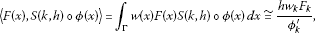(3.8)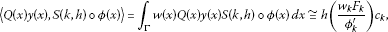(3.9)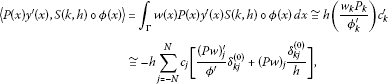(3.10)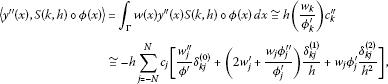(3.11)

where ${w}_{k}=w\left({x}_{k}\right)$. If we choose $h={\left(\pi d/\alpha N\right)}^{1/2}$ and $w\left(x\right)=1/{\varphi }^{\prime }\left(x\right)$ as given in  the accuracy for each equation between (3.8)-(3.11) will be $O\left({N}^{1/2}{e}^{-{\left(\pi d\alpha N\right)}^{1/2}}\right)$.

Using (3.5), (3.8)-(3.11), we obtain a linear system of equations for $2N+1$ numbers ${c}_{k}$.

The $2N+1$ linear system given in (3.5) can be expressed by means of matrices. Let $m=2N+1$, and let ${S}_{m}$ and ${c}_{m}$ be a column vector defined by

${S}_{m}\left(x\right)=\left(\begin{array}{c}{S}_{-N}\\ {S}_{-N+1}\\ ⋮\\ {S}_{N}\end{array}\right),\phantom{\rule{2em}{0ex}}{c}_{m}=\left(\begin{array}{c}{c}_{-N}\\ {c}_{-N+1}\\ ⋮\\ {c}_{N}\end{array}\right).$
(3.12)

Let ${A}_{m}\left(y\right)$ denote a diagonal matrix whose diagonal elements are $y\left({x}_{-N}\right),y\left({x}_{-N+1}\right),\dots ,y\left({x}_{N}\right)$ and non-diagonal elements are zero, and also let ${I}_{m}^{\left(0\right)}$, ${I}_{m}^{\left(1\right)}$ and ${I}_{m}^{\left(2\right)}$ denote the matrices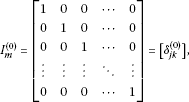(3.13)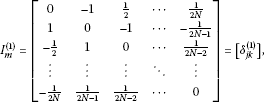(3.14)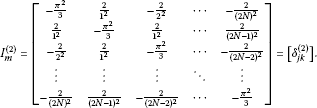(3.15)

With these notations, the discrete system of equations in (3.5) takes the form:(3.16)

Theorem 3.1 Let c be an m-vector whose jth component is ${c}_{j}$. Then the system (3.16) yields the following matrix system, the dimensions of which are $\left(2N+1\right)×\left(2N+1\right)$:

$\mathrm{\Phi }\cdot c={A}_{m}\frac{Fw}{{\varphi }^{\prime }}.$
(3.17)

Now we have a linear system of $\left(2N+1\right)$ equations of the $\left(2N+1\right)$ unknown coefficients. If we solve (3.17) by using LU or QR decomposition methods, we can obtain ${c}_{j}$ coefficients for the approximate sinc-Galerkin solution

$y\left(x\right)\approx {y}_{N}\left(x\right)=\sum _{k=-N}^{N}{c}_{k}S\left(k,h\right)\circ \varphi \left(x\right).$
(3.18)

## 4 Examples

Three examples were given in order to illustrate the performance of the sinc-Galerkin method to solve a singular Dirichlet-type boundary value problem in this section. The discrete sinc system defined by (3.18) was used to compute the coefficients ${c}_{j}$; $j=-N,\dots ,N$ for each example. All of the computations were done by an algorithm which we have developed for the sinc-Galerkin method. The algorithm automatically compares the sinc-method with the exact solutions. It is shown in Tables 2-4 and Figures 5-7 that the sinc-Galerkin method is a very efficient and powerful tool to solve singular Dirichlet-type boundary value problems.

Example 4.1 Consider the following singular Dirichlet-type boundary value problem on the interval $\left[0,1\right]$:

$\begin{array}{r}\frac{{d}^{2}}{d{x}^{2}}y\left(x\right)+\frac{y\left(x\right)}{x\left(x-1\right)}=-\frac{72}{1,045}{x}^{2}+\frac{12}{1,045}{x}^{3}+\frac{1}{209}{x}^{4}+1/19{x}^{5},\\ y\left(0\right)=0,\phantom{\rule{2em}{0ex}}y\left(1\right)=0.\end{array}$
(4.1)

The exact solution of (4.1) is

$\begin{array}{rcl}y\left(x\right)& =& \frac{1,834,592}{887,331,445}x+\frac{917,296}{887,331,445}{x}^{2}+\frac{458,648}{887,331,445}{x}^{3}-\frac{188,072}{34,571,355}{x}^{4}\\ +\frac{1,4131}{29,252,685}{x}^{5}+\frac{32}{278,597}{x}^{6}+\frac{1}{817}{x}^{7}.\end{array}$

We choose the weight function according to , $\varphi \left(x\right)=ln\left(\frac{1}{1-x}\right)$, $w\left(x\right)=\frac{1}{{\varphi }^{\prime }\left(x\right)}$, and by taking $d=\pi /2$, $h=\frac{2}{\sqrt{N}}$, ${x}_{k}=\frac{{e}^{kh}}{1+{e}^{kh}}$ for $N=8,16,32,100$, the solutions inFigure 5 and Table 2 are achieved.

Example 4.2 Let us have the following form of a singular Dirichlet-type boundary value problem on the interval $\left[0,1\right]$:

$\begin{array}{r}\frac{{d}^{2}}{d{x}^{2}}y\left(x\right)-\frac{1}{x}\frac{d}{dx}y\left(x\right)+\frac{y\left(x\right)}{x\left(x+1\right)}=-{x}^{3},\\ y\left(0\right)=0,\phantom{\rule{2em}{0ex}}y\left(1\right)=0.\end{array}$
(4.2)

The problem has an exact solution like

$\begin{array}{rcl}y\left(x\right)& =& \frac{1}{144}\cdot \left(14ln\left(x+1\right)x+14ln\left(x+1\right)-14x+6{x}^{2}-12{x}^{2}ln\left(2\right)\\ -2{x}^{3}+4{x}^{3}ln\left(2\right)+{x}^{4}-2{x}^{4}ln\left(2\right)+9{x}^{5}-18{x}^{5}ln\left(2\right)\right)/\left(-1+2ln\left(2\right)\right),\end{array}$

where $\varphi \left(x\right)=ln\left(\frac{1}{1-x}\right)$, $w\left(x\right)=\frac{1}{{\varphi }^{\prime }\left(x\right)}$.By taking $d=\pi /2$, $h=\frac{2}{\sqrt{N}}$, ${x}_{k}=\frac{{e}^{kh}}{1+{e}^{kh}}$ for $N=8,16,32,100$,we get the solutions in Figure 6 and Table 3.

Example 4.3 The following problem is given on the interval $\left[-1,4\right]$:

$\begin{array}{r}\frac{{d}^{2}}{d{x}^{2}}y\left(x\right)-\frac{d}{dx}y\left(x\right)=-\left(x-4\right),\\ y\left(-1\right)=0,\phantom{\rule{2em}{0ex}}y\left(4\right)=0,\end{array}$
(4.3)

where the exact solution of (4.3) is $y\left(x\right)=\frac{{x}^{2}{\mathrm{e}}^{4}-{x}^{2}{\mathrm{e}}^{-1}+15{\mathrm{e}}^{x}-6x{\mathrm{e}}^{4}+6x{\mathrm{e}}^{-1}-7{\mathrm{e}}^{4}-8{\mathrm{e}}^{-1}}{{\mathrm{2}\left(\mathrm{e}}^{4}-{\mathrm{e}}^{-1}\right)}$.

In this case, $\varphi \left(x\right)=ln\left(\frac{x+1}{4-x}\right)$, $w\left(x\right)=\frac{1}{{\varphi }^{\prime }\left(x\right)}$, and by taking $d=\pi /2$, $h=\frac{2}{\sqrt{N}}$, ${x}_{k}=\frac{-1+4{e}^{kh}}{1+{e}^{kh}}$ for $N=8,16,32,100$, we get results in Figure 7 and Table 4 .

## 5 Conclusion

The sinc-Galerkin method was employed to find the solutions of second-order Dirichlet-type boundary value problems on some closed real interval. The main purpose was to find the solution of boundary value problems which arise from the singular problems. The examples show that the accuracy improves with increasing number of sinc grid points N. We have also developed a very efficient and rapid algorithm to solve second-order Dirichlet-type BVPs with the sinc-Galerkin method on the Maple computer algebra system. All of the above computations and graphical representations were prepared by using Maple.

We give the Maple code in the Appendix section.

## Appendix: Maple code which we developed for the sinc-Galerkin approximation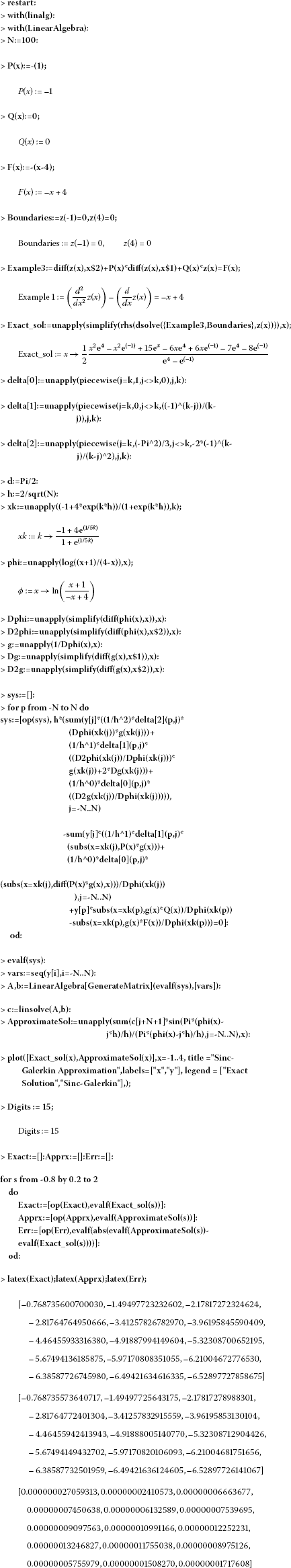## References

1. Stenger F: Approximations via Whittaker’s cardinal function. J. Approx. Theory 1976, 17: 222-240. 10.1016/0021-9045(76)90086-1

2. Stenger F: A sinc-Galerkin method of solution of boundary value problems. Math. Comput. 1979, 33: 85-109.

3. Whittaker ET: On the functions which are represented by the expansions of the interpolation theory. Proc. R. Soc. Edinb. 1915, 35: 181-194.

4. Whittaker JM Cambridge Tracts in Mathematics and Mathematical Physics 33. In Interpolation Function Theory. Cambridge University Press, London; 1935.

5. Lund J: Symmetrization of the sinc-Galerkin method for boundary value problems. Math. Comput. 1986, 47: 571-588. 10.1090/S0025-5718-1986-0856703-9

6. Lund J, Bowers KL: Sinc Methods for Quadrature and Differential Equations. SIAM, Philadelphia; 1992.

7. Lewis DL, Lund J, Bowers KL: The space-time sinc-Galerkin method for parabolic problems. Int. J. Numer. Methods Eng. 1987, 24: 1629-1644. 10.1002/nme.1620240903

8. McArthur KM, Bowers KL, Lund J: Numerical implementation of the sinc-Galerkin method for second-order hyperbolic equations. Numer. Methods Partial Differ. Equ. 1987, 3: 169-185. 10.1002/num.1690030303

9. Bowers KL, Lund J: Numerical solution of singular Poisson problems via the sinc-Galerkin method. SIAM J. Numer. Anal. 1987, 24(1):36-51. 10.1137/0724004

10. Lund J, Bowers KL, McArthur KM: Symmetrization of the sinc-Galerkin method with block techniques for elliptic equations. IMA J. Numer. Anal. 1989, 9: 29-46. 10.1093/imanum/9.1.29

11. Lybeck, NJ: Sinc domain decomposition methods for elliptic problems. PhD thesis, Montana State University, Bozeman, Montana (1994)

12. Lybeck NJ, Bowers KL: Domain decomposition in conjunction with sinc methods for Poisson’s equation. Numer. Methods Partial Differ. Equ. 1996, 12: 461-487. 10.1002/(SICI)1098-2426(199607)12:4<461::AID-NUM4>3.0.CO;2-K

13. Morlet AC, Lybeck NJ, Bowers KL: The Schwarz alternating sinc domain decomposition method. Appl. Numer. Math. 1997, 25: 461-483. 10.1016/S0168-9274(97)00068-8

14. Morlet AC, Lybeck NJ, Bowers KL: Convergence of the sinc overlapping domain decomposition method. Appl. Math. Comput. 1999, 98: 209-227. 10.1016/S0096-3003(97)10168-0

15. Alonso N, Bowers KL: An alternating-direction sinc-Galerkin method for elliptic problems. J. Complex. 2009, 25: 237-252. 10.1016/j.jco.2009.02.006

16. Ng M: Fast iterative methods for symmetric sinc-Galerkin systems. IMA J. Numer. Anal. 1999, 19: 357-373. 10.1093/imanum/19.3.357

17. Ng M, Bai Z: A hybrid preconditioner of banded matrix approximation and alternating-direction implicit iteration for symmetric sinc-Galerkin linear systems. Linear Algebra Appl. 2003, 366: 317-335.

18. Stenger F: Numerical Methods Based on Sinc and Analytic Functions. Springer, New York; 1993.

19. Koonprasert, S: The sinc-Galerkin method for problems in oceanography. PhD thesis, Montana State University, Bozeman, Montana (2003)

20. McArthur KM, Bowers KL, Lund J: The sinc method in multiple space dimensions: model problems. Numer. Math. 1990, 56: 789-816.

21. Stenger F: Numerical methods based on Whittaker cardinal, or sinc functions. SIAM Rev. 1981, 23: 165-224. 10.1137/1023037

22. Stenger F: Summary of sinc numerical methods. J. Comput. Appl. Math. 2000, 121: 379-420. 10.1016/S0377-0427(00)00348-4

23. Stenger F, O’Reilly MJ: Computing solutions to medical problems via sinc convolution. IEEE Trans. Autom. Control 1998, 43: 843. 10.1109/9.679023

24. Narasimhan S, Majdalani J, Stenger F: A first step in applying the sinc collocation method to the nonlinear Navier Stokes equations. Numer. Heat Transf., Part B 2002, 41: 447-462. 10.1080/104077902753725902

25. Mueller JL, Shores TS: A new sinc-Galerkin method for convection-diffusion equations with mixed boundary conditions. Comput. Math. Appl. 2004, 47: 803-822. 10.1016/S0898-1221(04)90066-1

26. El-Gamel M, Behiry SH, Hashish H: Numerical method for the solution of special nonlinear fourth-order boundary value problems. Appl. Math. Comput. 2003, 145: 717-734. 10.1016/S0096-3003(03)00269-8

27. Lybeck NJ, Bowers KL: Sinc methods for domain decomposition. Appl. Math. Comput. 1996, 75: 4-13.

## Author information

Authors

### Corresponding author

Correspondence to Aydin Secer.

### Competing interests

The authors declare that they have no competing interests.

### Authors’ contributions

AS proposed main idea of the solution schema by using Sinc Method for linear BVPs. He developed computer algorithm and worked on theoretical aspect of problem. MK searched the materials about study and compared with other techniques, contributed with his experience on Nonlinear Approximation methods.

## Authors’ original submitted files for images

Below are the links to the authors’ original submitted files for images.

## Rights and permissions

Reprints and Permissions

Secer, A., Kurulay, M. The sinc-Galerkin method and its applications on singular Dirichlet-type boundary value problems. Bound Value Probl 2012, 126 (2012). https://doi.org/10.1186/1687-2770-2012-126

• Accepted:

• Published:

• DOI: https://doi.org/10.1186/1687-2770-2012-126

### Keywords

• sinc-Galerkin method
• sinc basis functions
• Dirichlet-type boundary value problems
• LU decomposition method